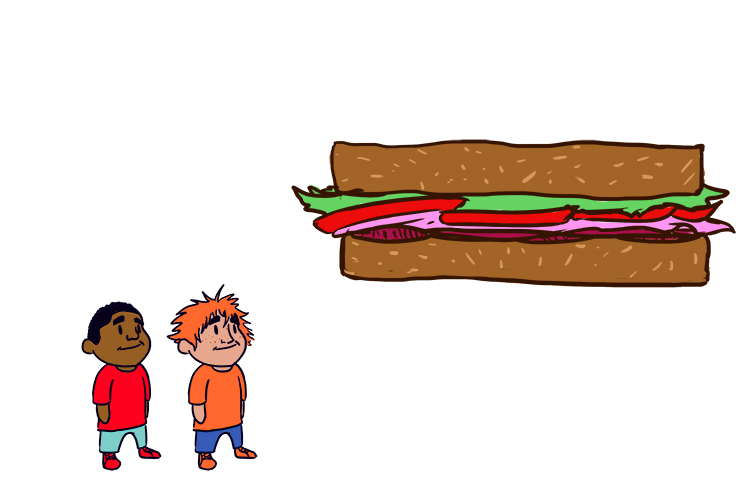# Proportion in maths (not the same as English language definition)

Proportion is comparing two ratios.

Proportion is putting an equals sign between two ratios.What proportion of this sandwich do you want? It looks like an equals sign so shall we have an equal amount each.

Example 1

If 8 apples cost £4.00 a total of 24 apples would cost £12

This can be expressed as follows:

8:4=24:12

or   8/4=24/12